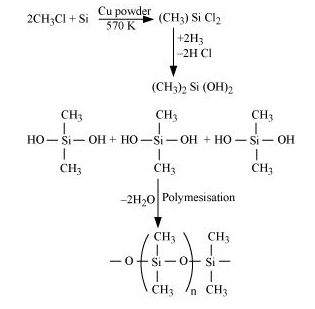# Explain the following reactions

Question:

Explain the following reactions

(a) Silicon is heated with methyl chloride at high temperature in the presence of copper;

(b) Silicon dioxide is treated with hydrogen fluoride;

(c) CO is heated with ZnO;

(d) Hydrated alumina is treated with aqueous NaOH solution.

Solution:

(a) When silicon reacts with methyl chloride in the presence of copper (catalyst) and at a temperature of about $537 \mathrm{~K}$, a class of organosilicon polymers called methylsubstituted chlorosilanes $\left(\mathrm{MeSiCl}_{3}, \mathrm{Me}_{2} \mathrm{SiCl}_{2}, \mathrm{Me}_{3} \mathrm{SiCl}\right.$, and $\mathrm{Me}_{4} \mathrm{Si}$ ) are formed.(b) When silicon dioxide $\left(\mathrm{SiO}_{2}\right)$ is heated with hydrogen fluoride $(\mathrm{HF})$, it forms silicon tetrafluoride $\left(\mathrm{SiF}_{4}\right)$. Usually, the Si-O bond is a strong bond and it resists any attack by halogens and most acids, even at a high temperature. However, it is attacked by HF.

$\mathrm{SiO}_{2}+4 \mathrm{HF} \longrightarrow \mathrm{SiF}_{4}+2 \mathrm{H}_{2} \mathrm{O}$

The $\mathrm{SiF}_{4}$ formed in this reaction can further react with $\mathrm{HF}$ to form hydrofluorosilicic acid.

$\mathrm{SiF}_{4}+2 \mathrm{HF} \longrightarrow \mathrm{H}_{2} \mathrm{SiF}_{6}$

(c) When CO reacts with ZnO, it reduces ZnO to Zn. CO acts as a reducing agent.

$\mathrm{ZnO}_{(s)}+\mathrm{CO}_{(g)} \stackrel{\Delta}{\longrightarrow} \mathrm{Zn}_{(s)}+\mathrm{CO}_{2(g)}$

(d) When hydrated alumina is added to sodium hydroxide, the former dissolves in the latter because of the formation of sodium meta-aluminate.

$\mathrm{Al}_{2} \mathrm{O}_{3} \cdot 2 \mathrm{H}_{2} \mathrm{O}+2 \mathrm{NaOH} \longrightarrow 2 \mathrm{NaAlO}_{2}+3 \mathrm{H}_{2} \mathrm{O}$